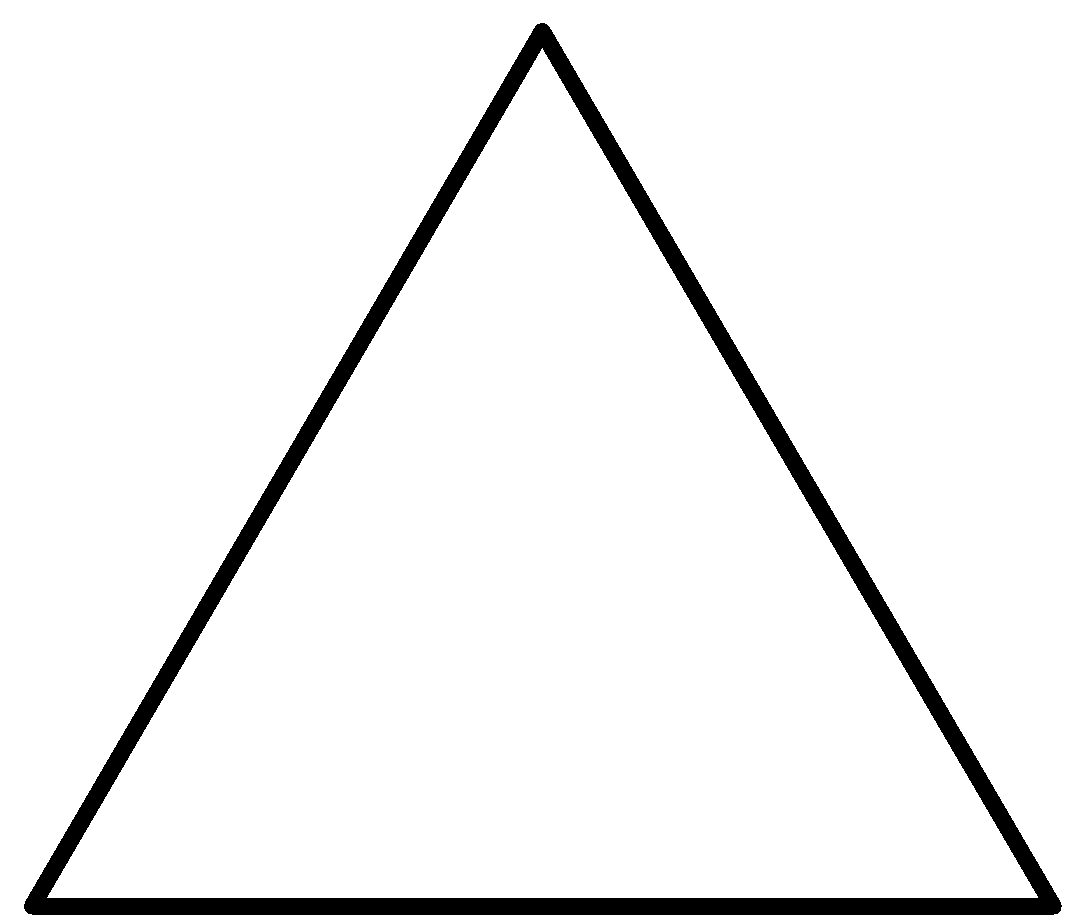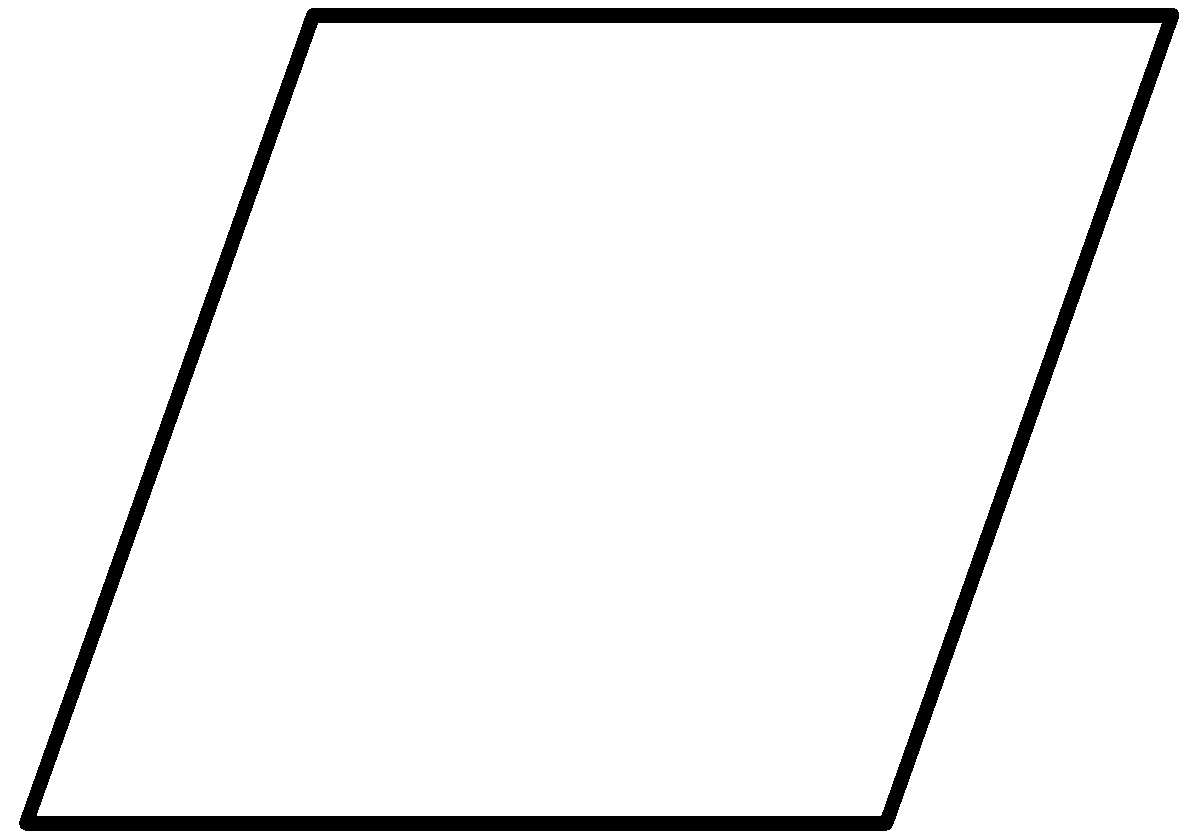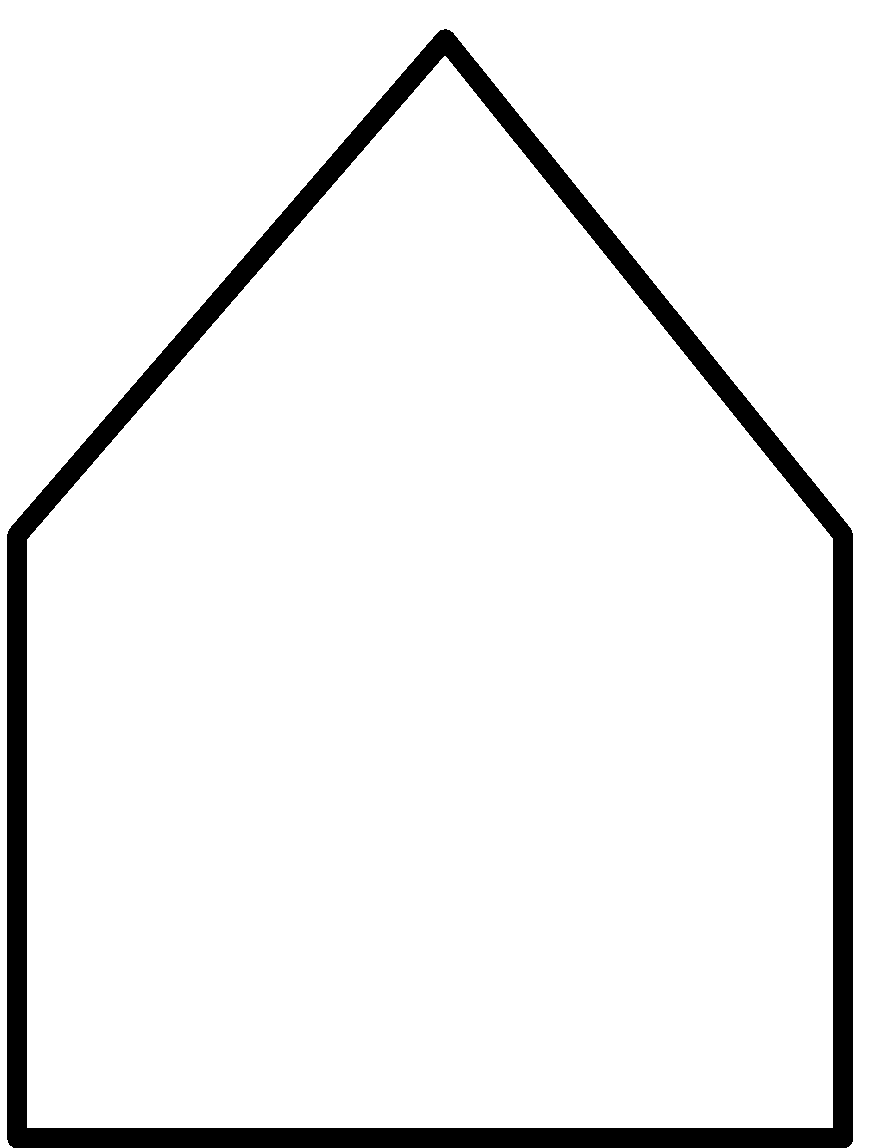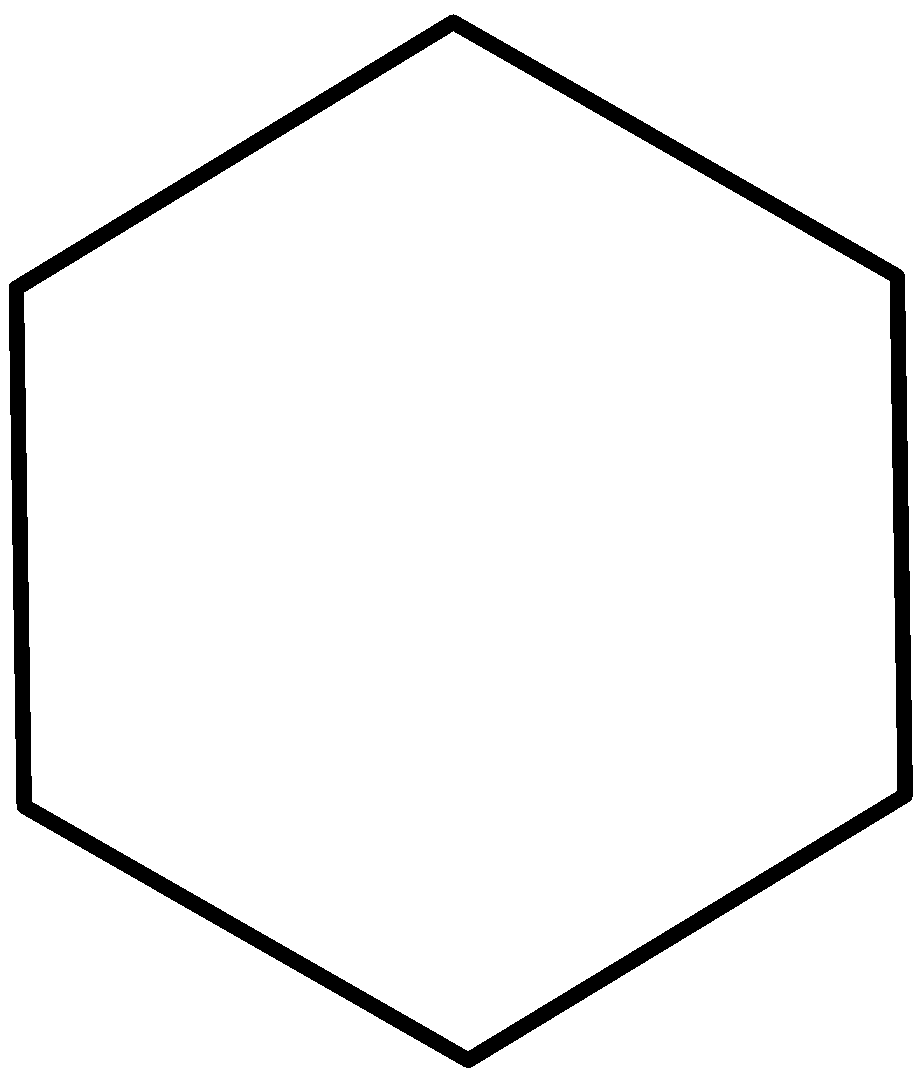## POLYGON:

A polygon is a closed figure made by connecting line segments, where each line segment end connects to only one end of two other line segments.

A polygon is traditionally a plane figure that is bounded by a closed path, composed of a finite sequence of straight line segments. These segments are called its edges or sides, and the points where two edges meet are the polygon's vertices (singular: vertex) or corners. An n-gon is a polygon with n sides. The interior of the polygon is sometimes called its body. A polygon is a 2-dimensional example of the more general polytope in any number of dimensions.The line segments forming a polygon are called its sides and the end-points of the line segments are called its vertices. In other words, the meeting point of a pair of sides is called a vertex of the polygon.In figure (i), ABC is a polygon having three sides AB, BC and CA. Points A, B, C are known as the vertices. As the figure consist of three sides. So, it is called a triangle.

Figure (ii) is a polygon with four sides AB, BC, CD and DA. It has four vertices A, B, C and D. Such a figure is called a quadrilateral. Line segments AC and BD are known as the diagonals.

A closed figure formed by five line segments is known as a pentagon. Figure (iii) is a pentagon with AB, BC, CD, DE and EA as five sides. A, B, C, D and E are five vertices of the pentagon. Line segments AC, AD, BD and BE are diagonals.

Figure (v) is not a polygon as BC is not a line segment.

### CLASSIFICATION OF POLYGONS

Polygons are classified according to the number of sides they have.

 Name No. of sides Figures Triangle 3Quadrilateral 4Pentagon 5Hexagon 6Heptagon 7Octagon 8Nonagon 9NCERT solutions for class 8 Maths  prepared by Physics Wallah will help you to solve your NCERT text book exercise.